# Chapter 24 Comparing Means Comparing Two Means l

• Slides: 48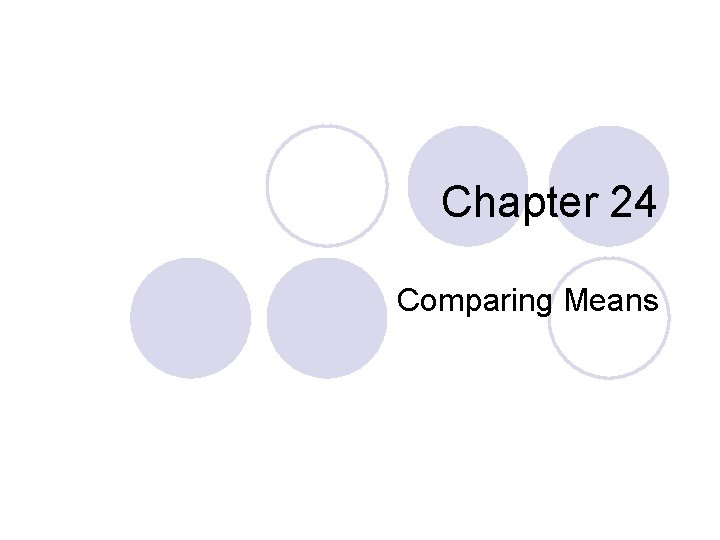Chapter 24 Comparing MeansComparing Two Means l An educator believes that new reading activities for elementary school children will improve reading comprehension scores. She randomly assigns her third-grade students to one of two groups. The first group will use a traditional reading program and the second group will use the new reading activities. At the end of the experiment, both groups take a reading comprehension exam. Are the scores for the new reading activities group higher than for the traditional group?Comparing Two Means l Look at boxplot of each group’s scores.Comparing Two Means l What do you see? ¡ ____________________________________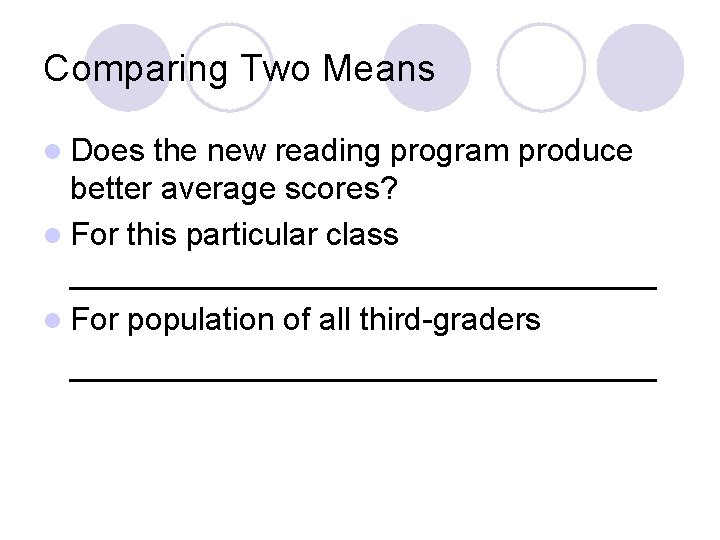Comparing Two Means l Does the new reading program produce better average scores? l For this particular class _________________ l For population of all third-graders _________________Comparing Two Means l μ 1 = _______________ l μ 2 = _______________ l Interested in quantity μ 1 - μ 2.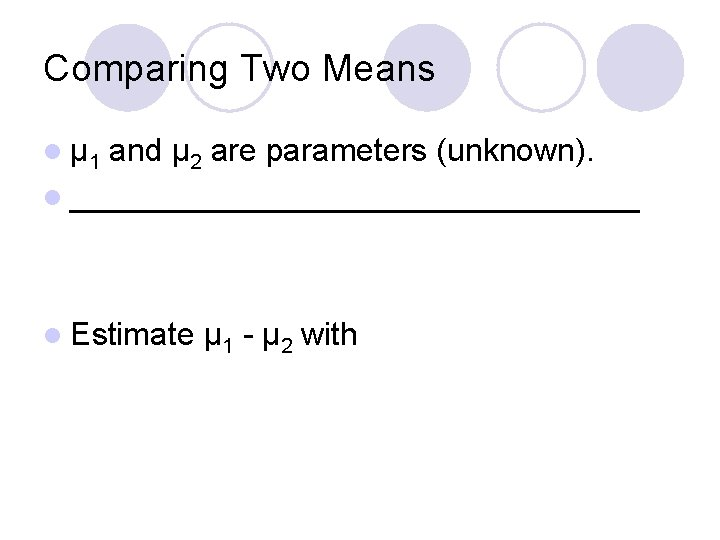Comparing Two Means l μ 1 and μ 2 are parameters (unknown). l ________________ l Estimate μ 1 - μ 2 withSampling Distribution l Assumptions: ¡ Random Samples ¡ Samples are Independent ¡ Nearly Normal Population DistributionsSampling Distribution l If is Assumptions hold, sampling distribution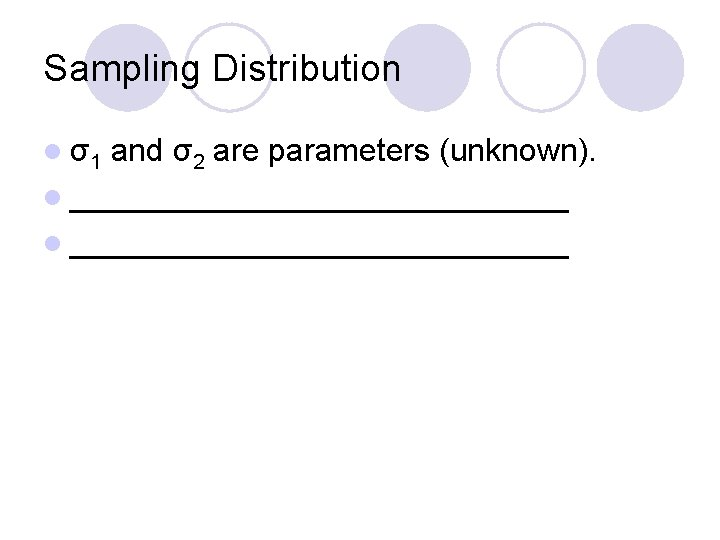Sampling Distribution l σ1 and σ2 are parameters (unknown). l ____________________________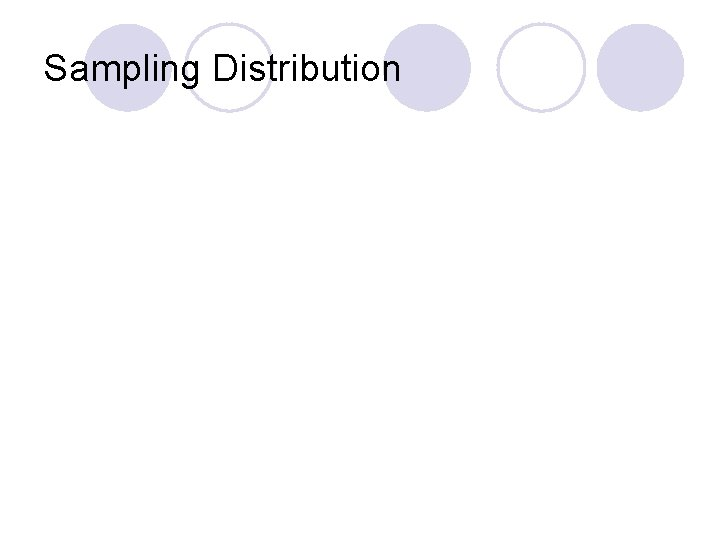Sampling DistributionDegrees of Freedom? lt really doesn’t have a t distribution. l The true distribution of t is ________________ when you use this formula for the degrees of freedom.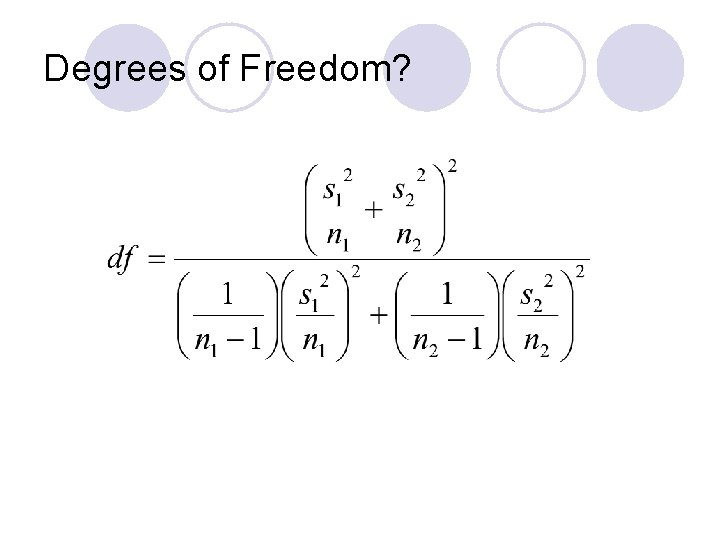Degrees of Freedom?Degrees of Freedom? l Problem: ¡ ____________________________________ l Can use simpler, more conservative method. ¡ __________________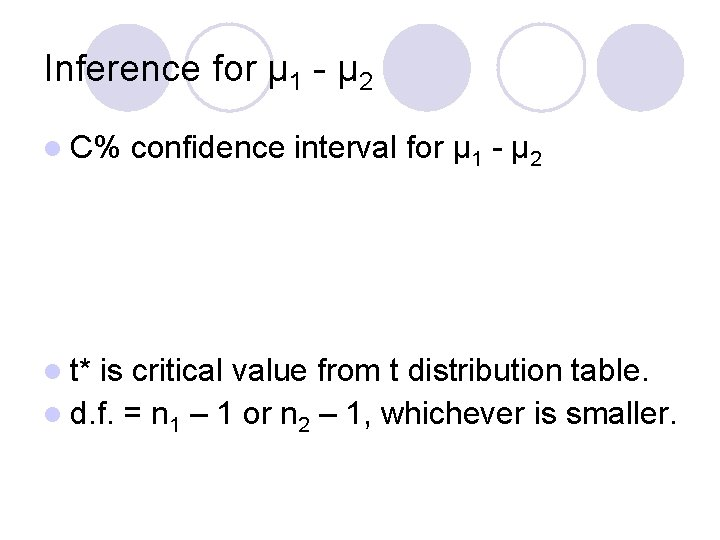Inference for μ 1 - μ 2 l C% l t* confidence interval for μ 1 - μ 2 is critical value from t distribution table. l d. f. = n 1 – 1 or n 2 – 1, whichever is smaller.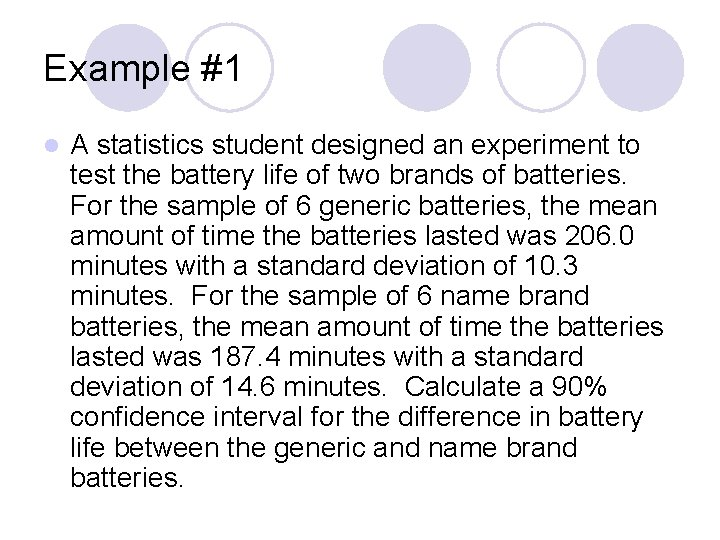Example #1 l A statistics student designed an experiment to test the battery life of two brands of batteries. For the sample of 6 generic batteries, the mean amount of time the batteries lasted was 206. 0 minutes with a standard deviation of 10. 3 minutes. For the sample of 6 name brand batteries, the mean amount of time the batteries lasted was 187. 4 minutes with a standard deviation of 14. 6 minutes. Calculate a 90% confidence interval for the difference in battery life between the generic and name brand batteries.Example #1 (cont. ) l Assumptions: ¡ Random l samples OK ¡ Independent l different batteries for each sample. ¡ Nearly l samples Normal data shows no real outliers.Example #1 (cont. )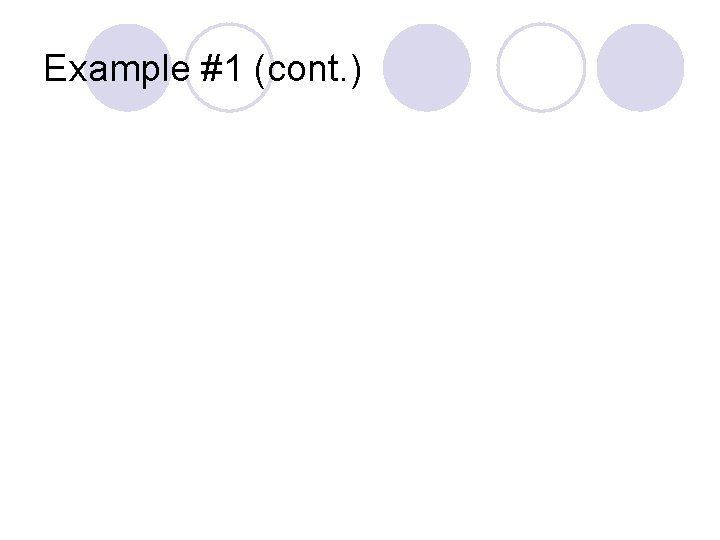Example #1 (cont. )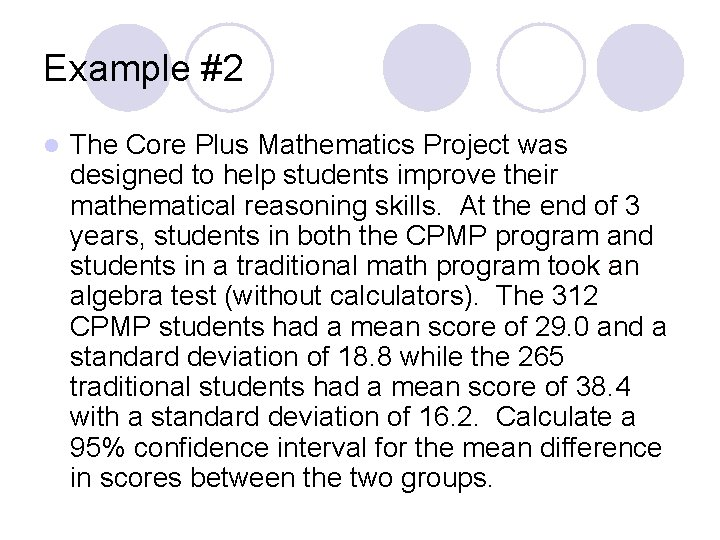Example #2 l The Core Plus Mathematics Project was designed to help students improve their mathematical reasoning skills. At the end of 3 years, students in both the CPMP program and students in a traditional math program took an algebra test (without calculators). The 312 CPMP students had a mean score of 29. 0 and a standard deviation of 18. 8 while the 265 traditional students had a mean score of 38. 4 with a standard deviation of 16. 2. Calculate a 95% confidence interval for the mean difference in scores between the two groups.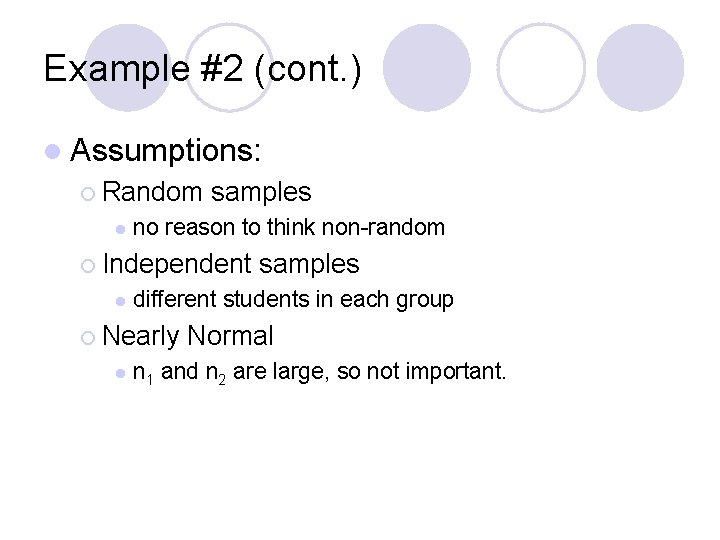Example #2 (cont. ) l Assumptions: ¡ Random l samples no reason to think non-random ¡ Independent l different students in each group ¡ Nearly l samples Normal n 1 and n 2 are large, so not important.Example #2 (cont. ) l d. f. = smaller of 311 and 264 = 264 l μ 1 = _______________ l μ 2 = _______________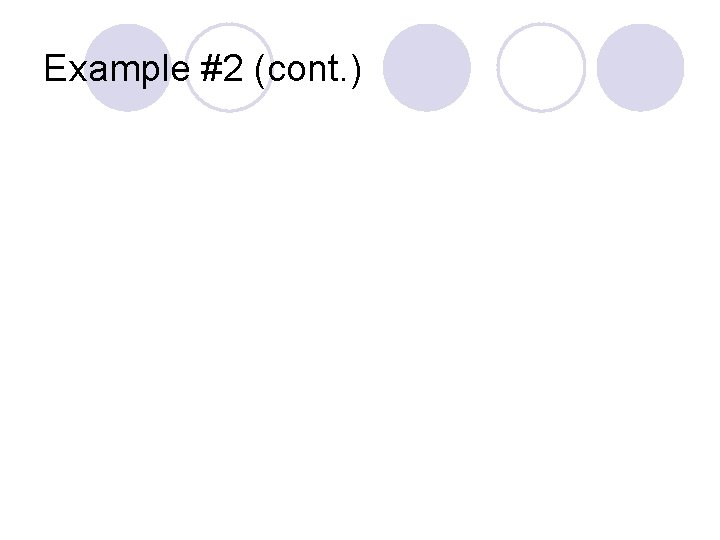Example #2 (cont. )Example #2 (cont. )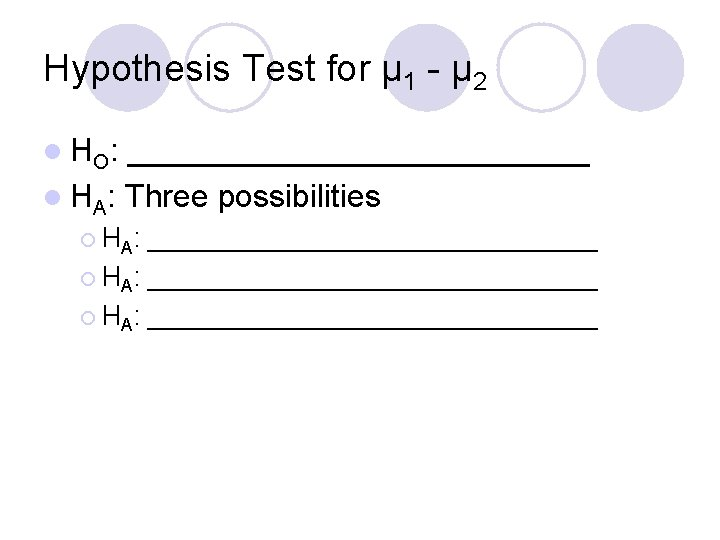Hypothesis Test for μ 1 - μ 2 l HO : _____________ l HA: Three possibilities ¡ HA : ______________________________ ¡ HA: _______________Hypothesis Test for μ 1 - μ 2 l Assumptions ¡ Random samples. ¡ Independent samples. ¡ Nearly Normal Population Distributions.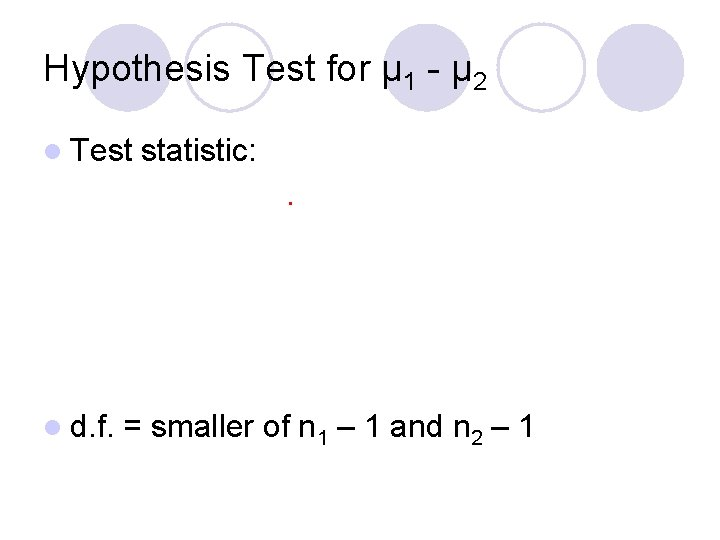Hypothesis Test for μ 1 - μ 2 l Test l d. f. statistic: = smaller of n 1 – 1 and n 2 – 1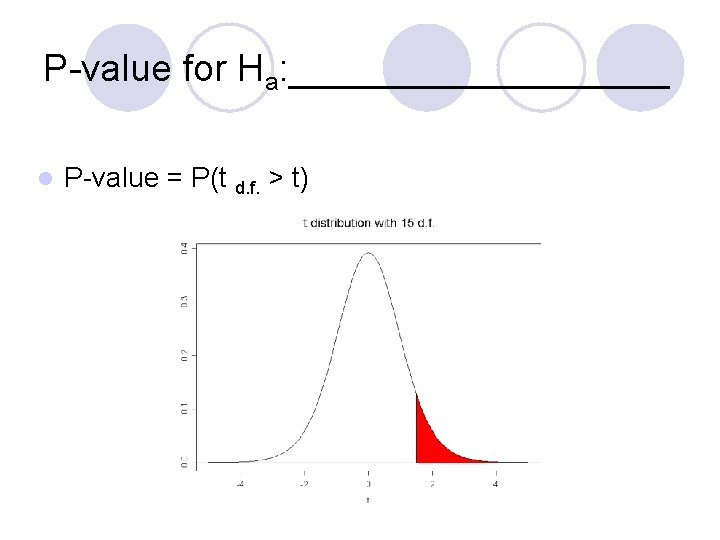P-value for Ha: _________ l P-value = P(t d. f. > t)P-value for Ha: _________ l P-value = P(t d. f. < t)P-value for Ha: _________ l P-value = 2*P(t d. f. > |t|)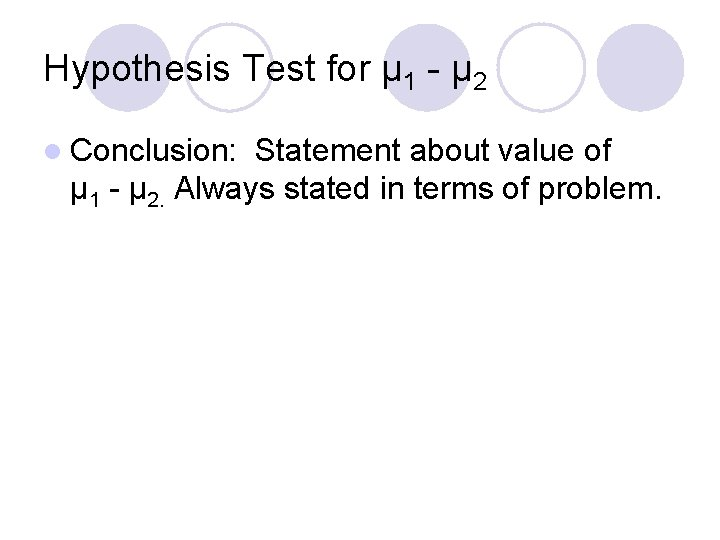Hypothesis Test for μ 1 - μ 2 l Conclusion: Statement about value of μ 1 - μ 2. Always stated in terms of problem.Example #1 l Back to the reading example. The educator takes a random sample of all third graders in a large school district and divides them into the two groups. The mean score of the 18 students in the new activities group was 51. 72 with a standard deviation of 11. 71. The mean score of the 20 students in the traditional group was 41. 8 with a standard deviation of 17. 45. Is this evidence that the students in the new activities group have a higher mean reading score? Use α = 0. 1.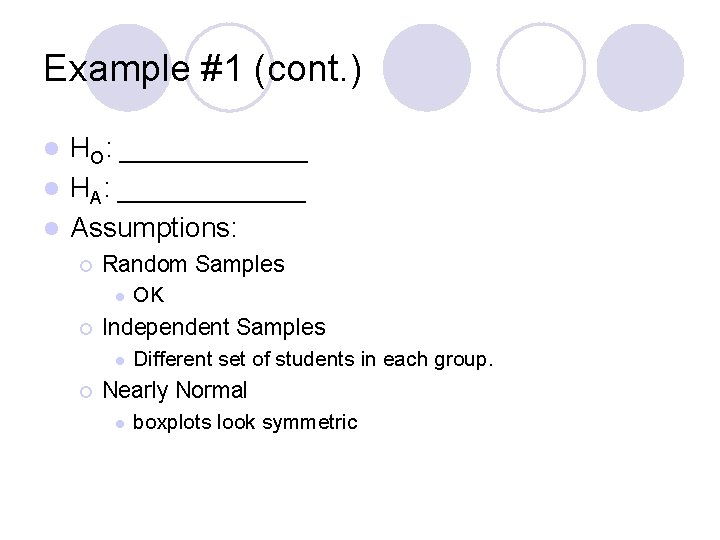Example #1 (cont. ) HO: ______ l HA: ______ l Assumptions: l ¡ Random Samples l ¡ Independent Samples l ¡ OK Different set of students in each group. Nearly Normal l boxplots look symmetricExample #1 (cont. )Example #1 (cont. ) d. f. = smaller of 17 or 19 = 17 l P-value l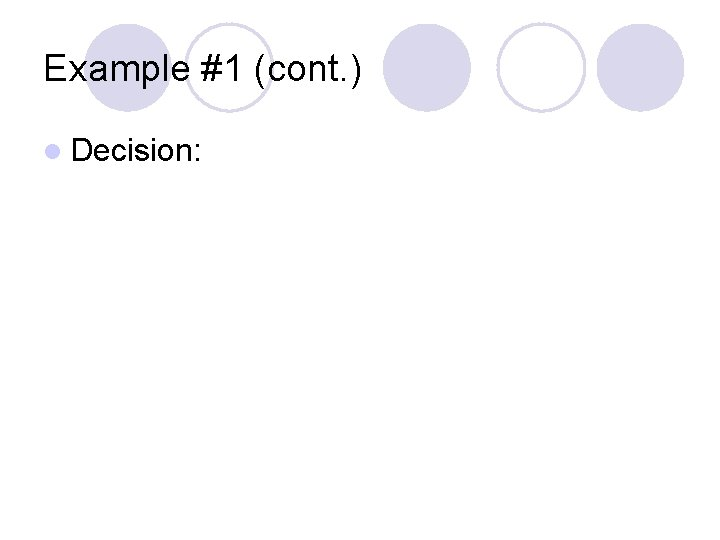Example #1 (cont. ) l Decision:Example #1 (cont. ) l Conclusion:Example #2 l In June 2002, the Journal of Applied Psychology reported on a study that examined whether the content of TV shows influenced the ability of viewers to recall brand names of items featured in commercials. Researchers randomly assigned volunteers to watch either a program with violent content or a program with neutral content. Both programs featured the same 9 commercials. After the shows ended, subjects were asked to recall the brands in the commercials. Is there evidence that viewer memory for ads differs between programs? Use α = 0. 05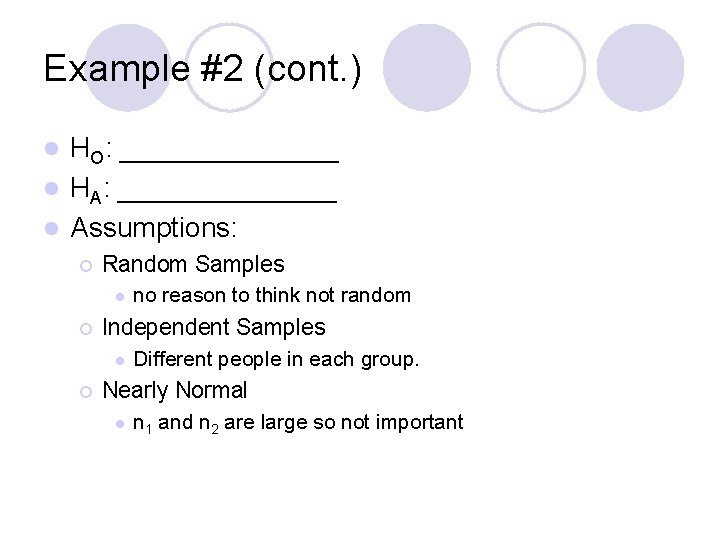Example #2 (cont. ) HO: _______ l HA: _______ l Assumptions: l ¡ Random Samples l ¡ Independent Samples l ¡ no reason to think not random Different people in each group. Nearly Normal l n 1 and n 2 are large so not importantExample #2 (cont. )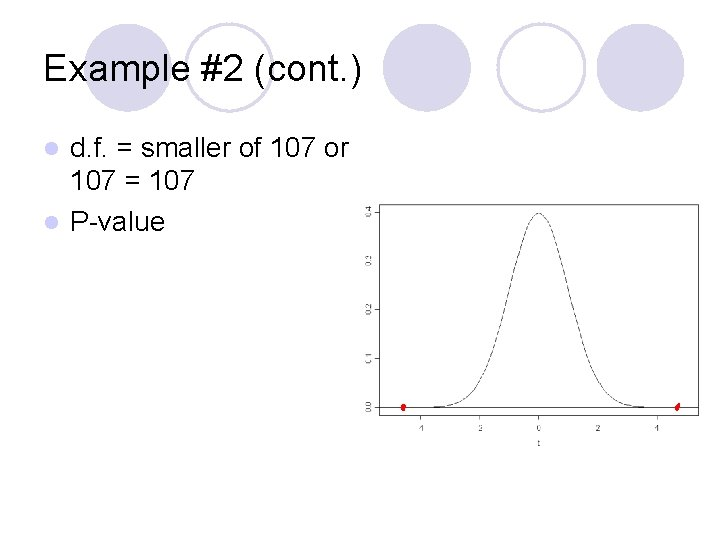Example #2 (cont. ) d. f. = smaller of 107 or 107 = 107 l P-value lExample #2 (cont. ) l Decision: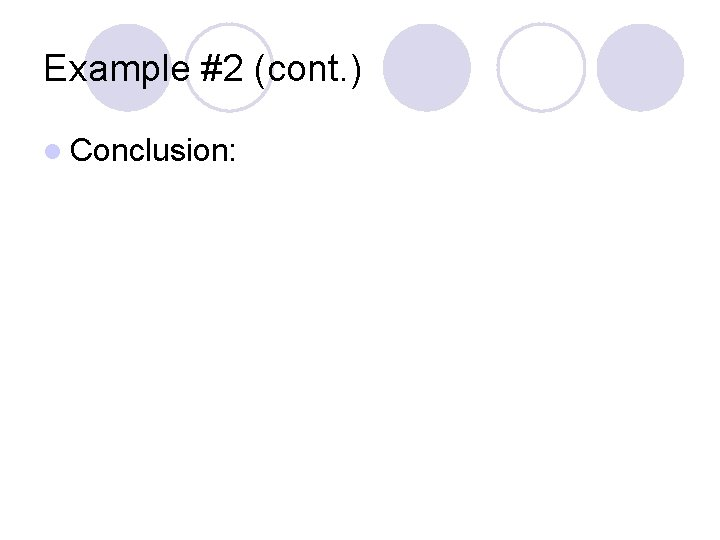Example #2 (cont. ) l Conclusion: